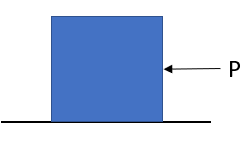## Block Speed

A 10-kg block is sliding along a frictionless surface due to a constant force P = 5 N. At what time (in seconds) will the block be moving at 25 m/s?Expand Hint
$$Force=mass\times acceleration$$$Hint 2 $$velocity=acceleration \times time$$$
Using Newton’s second law of motion:
$$Force=mass\times acceleration\rightarrow a=\frac{F}{m}$$$$$a=\frac{5N}{10kg}=0.5\:m/s^2$$$
Next,
$$velocity=acceleration \times time\rightarrow t=\frac{v}{a}$$$$$t=\frac{25m/s}{0.5m/s^2}=50\:seconds$$$
50 seconds
Similar Problems from FE Sub Section: Constant Mass

Similar Problems from FE Section: Particle Kinetics

Similar Problems from FE Section: Particle Kinematics